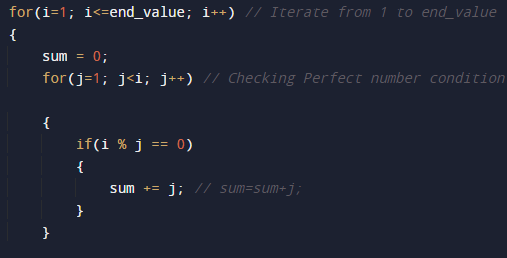C Program To Print all Perfect number between 1 and given number

In this tutorial you will learn about the C Program To Print all Perfect number between 1 and given number and its application with practical example.

In this tutorial, we will learn to create a C program that will find perfect number between  1 to entered number using C programming.

Prerequisites

Before starting with this tutorial we assume that you are best aware of the following C programming topics:

• C Operators.
• Basic input/output.
• C loop statements.
• Nested loop.
• Conditional statement.

What Is Perfect Number is?

Perfect number is a positive integer number,that equals to sum of its positive divisors not include the number itself is equal to that number.

Example

Divisor of  6 are 1,2 and 3. and sum of these  are (1+2+3=6). So we can say that 6 is a perfect number in which we will not induced 6 i.e exclude 6 .So number is  called perfect when divisor of number added and that create the number itself called Perfect number.

C Program To Print all Perfect number between 1 and given number.

In this program we will find that given number is perfect number or not using for loop.
Firstly we declare required header file and variable and also initiates required values in variable. Next we take value from user at run time and then after we will find that the given value is perfect number or not.

OutputIn the above program, we have first declared and initialized a set variables required in the program.

• end_value   =  holding given number
• sum              = adding all divisor.
• i                     = for iteration of a loop.
• j                     = for iteration of a loop.
Logic behind finding the perfect number is ..
Let : no = 6
Proper divisors of number 6 are 1, 2, 3.
Sum of all its proper divisors exclude 6 = 1 + 2 + 3 = 6.
Hence 6 is a perfect number.

Now run the  loop from 1 to end_value ,by  incrementing i with 1 in each iteration. The loop structure should look like thisand within the loop for each iteration printing the value of i if it is a Perfect number.So here is the program that  will find  1 to given number perfect number.

In this tutorial we have learn about the C Program To Print all Perfect number between 1 and given number and its application with practical example. I hope you will like this tutorial.